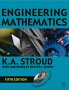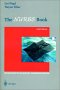# Maths - Books3D Math Primer - Aimed at complete beginners to vector and matrix algebra.Mathematics for 3D game Programming - Includes introduction to Vectors, Matrices, Transforms and Trigonometry. (But no euler angles or quaternions). Also includes ray tracing and some linear & rotational physics also collision detection (but not collision response).Engineering Mathematics - This book has been going for a long time and it is now in its 5th edition, so it is tried and tested.Schaum's Outline of Theory and Problems of Tensor Calculus - I'm finding this hard going, it starts off with as review of linear algebra, matrix notation, etc. It redefines a lot of conventions which are hard to relearn, such as superscrips instead of subscripts to identify elements, and a summation convention, then it goes into coordinate transformations. I cant find any definition of what a tensor is. I think this book is aimed at people who already have some knowledge of the subject.

### Books about Curves and SurfacesCurves and Surfaces in Geometric Modeling: Theory and AlgorithmsThe NURBs Book (Monographs in Visual Communication) - A NURBS book for software developer, it covers the theory and also the practical side.

Other books on this subject